Intelligent Automation & Soft ComputingDOI:10.32604/iasc.2022.018975Article

Design and Analysis of 4-bit 1.2GS/s Low Power CMOS Clocked Flash ADC

1Department of ECE, University College of Engineering, Ariyalur, 621705, India
2Department of ECE, Saranathan College of Engineering, Trichy, 620012, India
*Corresponding Author: G. Prathiba. Email: prathibagurusamy@gmail.com
Received: 28 March 2021; Accepted: 11 June 2021

Abstract: High-quality, high-resolution flash ADCs are used in reliable VLSI (Very Large-Scale Integrated) circuits to minimize the power consumption. An analogue electrical signal is converted into a discrete-valued sequence by these ADCs. This paper proposes a four-bit 1.2GS/s low-power Clocked Flash ADC (C-FADC). A low-power Clocked-Improved Threshold Inverter Quantization (CITIQ) comparator, an Adaptive Bubble Free (ABF) logic circuit, and a compact Binary Encoder (BE) are all part of the presented structure. A clock network in the comparator circuit reduces skew and jitters, while an ABF logic circuit detects and corrects fourth order bubble faults detected from thermometer code, and then the BE transforms the bubble free code into binary code. A Tanner EDA with 250-nm Technology is used to implement the C-FADC. The proposed design achieves ENOB of 3.56, uses 3.24 mW of power, and has a FOM of 0.274pJ/conv.-step at an input frequency of 85 MHz. The suggested C-FADC has differential and integral nonlinearities of ±0.65 LSB and +0.45/-0.5 LSB, respectively.

Keywords: Skew and jitter; offset voltage; clock network; adaptive bubble free logic

1  Introduction

This paper is organized as follows: The section1 introduces FADC and its features. The study foundation is mentioned in Section 2. Section 3 proposes a Clocked FADC built with the aid of a CITIQ comparator, an ABF, and a binary encoder. Graphical response and outcomes are given out in Section 4. Section 5 exhibits the concluding part.

2  Related Work

The area, proficient structure known as a multiplexer pass gate encoder and low power comparator architecture is coordinated by the R-Flash ADC structure. The sensitivity of the elements used in the framework and its adaptation to fault tolerance can be used to determine the system reliability. If a problem is found in the redundant block, the bubble fault corrector recognizes the error and ensures the system . The two phases of the clock (ON and OFF) operation is used in a time-dependent flash structured ADC . This two-phase plan significantly reduces power consumption. The input capacitance in this work is kept to a minimum, and an on-chip adjustment circuit is used to suppress various mismatches. Previous research in flash ADC revealed a low-resolution quality as a result of converting the thermometer code to parallel code, which limits the speed and power. Clocked Flash ADC is suggested to address these limitations.Figure 1: Blocks involved in C-Flash ADC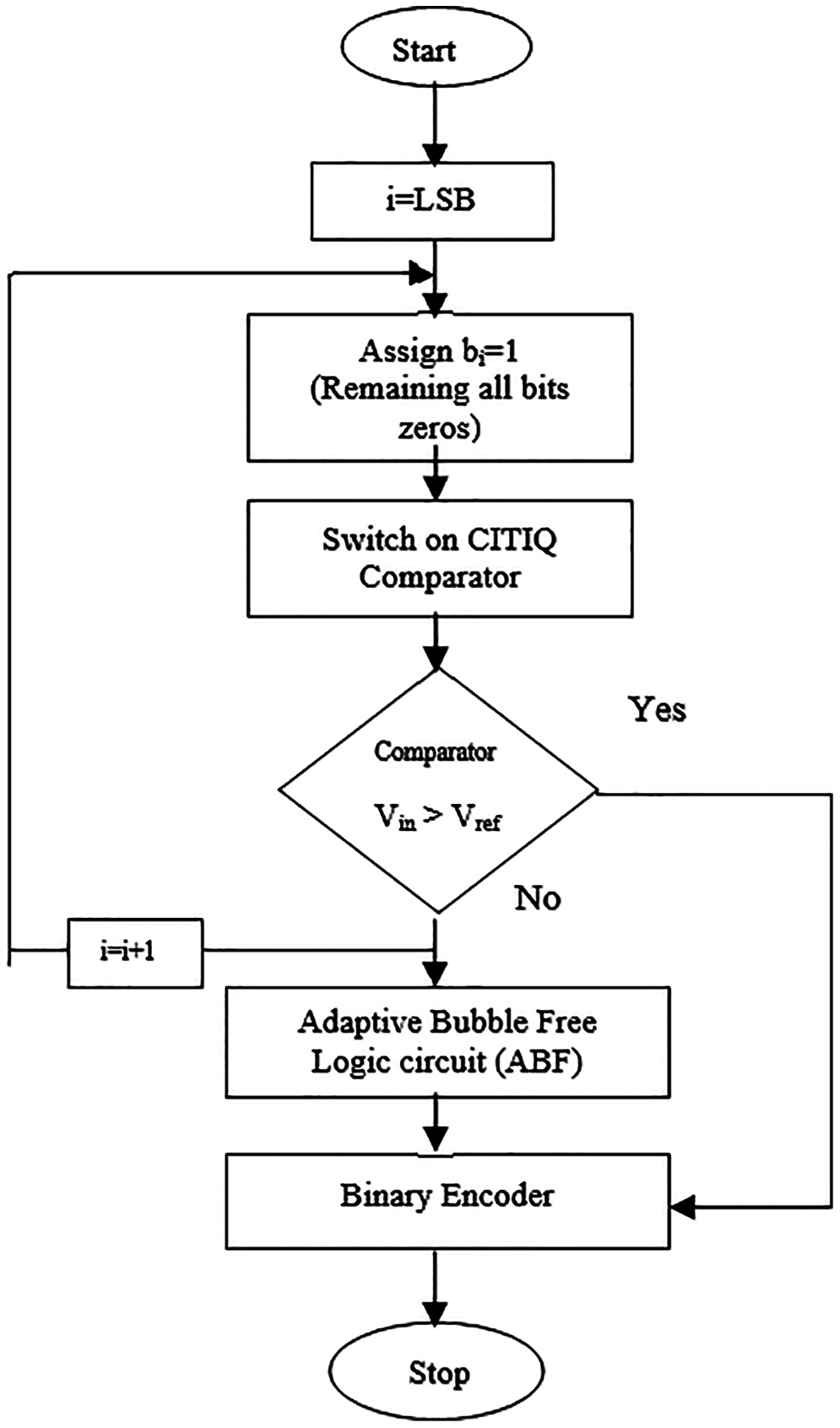Figure 2: Flow chart of proposed C-FADC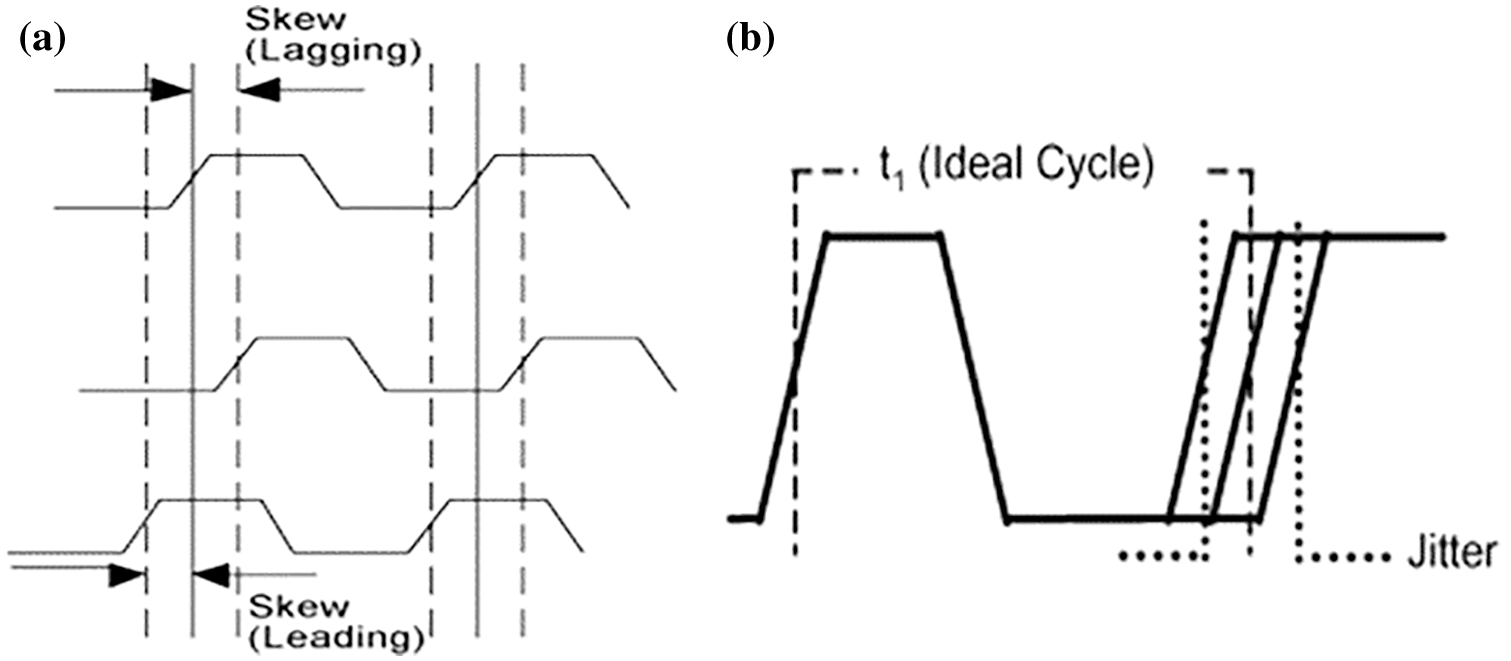Figure 3: (a) Clock skews (b) Jitter

3.1 Proposed CITIQ Comparator

A group of resistors from the ladder circuit is used to produce a comparator reference voltage in a typical flash ADC. The comparator must be large because the reference potential produced by the group of resistors in the circuit consumes more power. It can be overcome by CITIQ comparator technique. This paper proposes a CITIQ comparator with a small size and low power consumption. A CITIQ comparator, made up of two CMOS inverters and a dual set of NMOS and PMOS transistors, compares the analogue input potential to an internally generated reference potential and provide a binary output. Internal reference voltages are produced from the threshold voltage (Vt), eliminating the need for a resistor ladder circuit in the Flash ADC. The CITIQ comparator compares the input voltage to the internally created reference potential, which is resolved by changing the width of the transistors, which is the first stage of the cascading structure. Obtain sharper threshold and full output voltage swing by using the succeeding stage of the cascading structure called a gain booster.

The schematic view of the proposed single stage Clocked ITIQ comparator is shown in Fig. 4. It employs a pair of cascaded inverters with PMOS 1, PMOS 2, and the NMOS 1 as the ITIQ comparator, as well as two sets of the PMOS 4, NMOS 2, and PMOS 5, NMOS 3 transistors connected in parallel. The ITIQ comparator networks are pulled down and up by each pair. A clock pulse guides the pair of PMOS and NMOS transistors; the NMOS is linked to the clock, while the PMOS is linked to the clock bar. Internally, the CITIQ comparator generates a reference voltage; the length (L) and width (W) of PMOS and NMOS are varied to obtain the desired switching voltage. The comparator switching voltage is determined by the NMOS and PMOS threshold voltages. To reduce the design power consumption, the threshold voltage should not be deviated too far. In contrast, regardless of whether the transistor length is held very short to achieve a speed, channel noise can affect the output, resulting in a nonlinear switching pattern. As a result, the transistor length (L) is set at a nominal value, and the width of the transistor is used to generate different switching voltages. As the transistor width (W) is increased, the transistor current drive capability increases, reducing the signal rise or fall time at the gate terminal's output. The active area of active devices increases with increased transistor size and the layout area may increase. The mathematical Eqs. (1)–(4) used to choose these switching potentials are as follows:

Vs=μpWpμnWp.VAIR1+μpWpμnWp (1)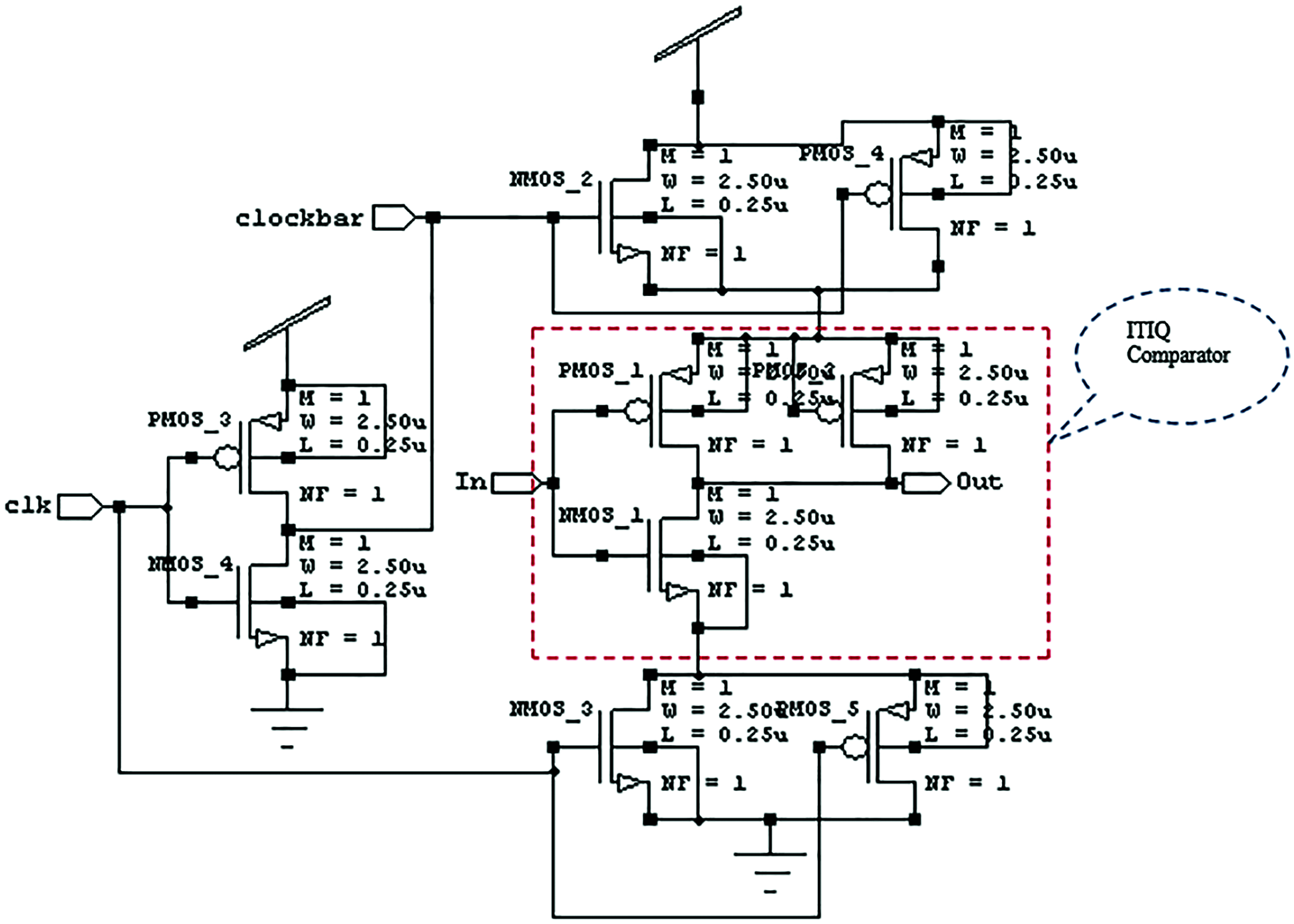Figure 4: Schematic view of clocked ITIQ comparator

VAIR=[(Vdd|Vtp|)+Vtn] (2)

Minimum size variation of transistor

Vs=Vdd2 (3)

The least significant bit (LSB for n-bit) voltage

LSB=VAIR2n1 (4)

where,

Wp is the PMOS transistor width

Wn is the NMOS transistor width

Vdd is the source potential

Vtn is the NMOS transistor threshold voltage

Vtp is the PMOS transistor threshold voltage

μn is the mobility of electron

μp is the mobility of hole

It is expected that the NMOS length (Ln) = PMOS length (Lp). The transistor parameters of CITIQ comparator are shown in Tab. 1. The switching voltage was determined by initially varying NMOS transistor sizes, (1.25–2.8 µm) and then maintaining a constant value (3 µm). Tab. 2 also shows the different sizes of PMOS transistors (0.21–3.72 m). Based on the comparator switching voltage, the LSB (Least Significant Bit) for n-bit is 0.106 V.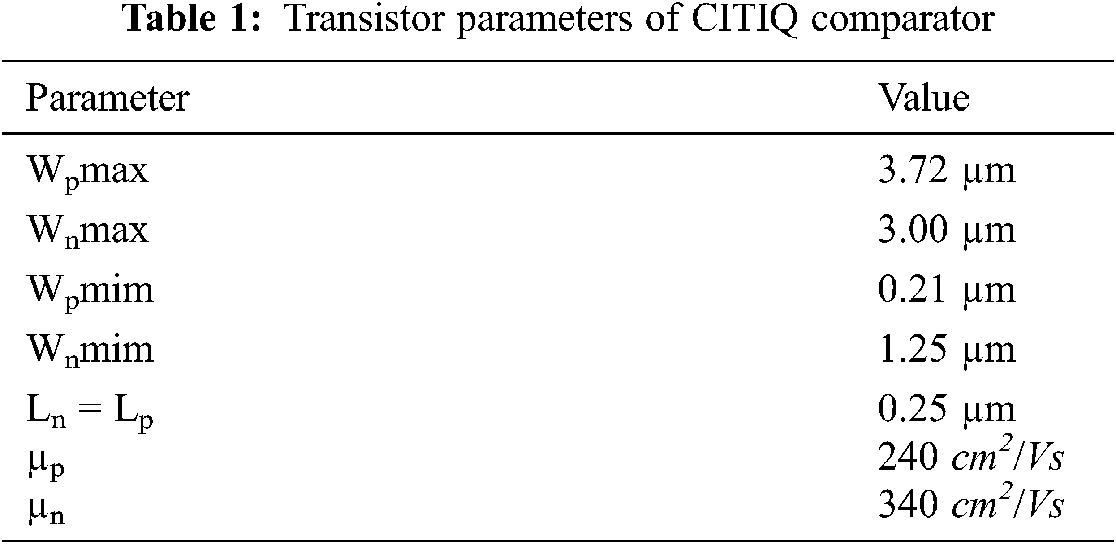3.2 Adaptive Bubble Free (ABF) Logic Circuit and Binary Encoder (BE)

The binary or parallel encoder is used to convert a 15-bit comparator output to a 4-bit digital word, with Adaptive Bubble Free (ABF) logic to eliminate bubble faults from the thermometer code. One or more bits of invalid code which be created by a bubble fault in the thermometer code. It is classified by the quantity of invalid 0 Bit between 1 Bits named as 1st order, 2nd order, 3rd order and 4th order bubble faults. The bubble-free circuitry has fifteen 3-input NAND logic gates and fifteen inverters and is inserted between the comparator and binary encoder. Eight 4-input NAND logic gates and four 2-input OR logic gates make up the binary encoder circuit. Fig. 5, shows the functional blocks of the Adaptive Bubble Free logic with Binary Encoder. The following Eqs. (5)–(8) illustrate the Boolean expression that relates the predicted binary output E3 –E0 obtained from the binary encoder using adaptive bubble free logic circuit.

E0=L1L3L5L7¯+L9L11L13L15¯ (5)

E1=L2L3L6L7¯+L10L11L14L15¯ (6)

E2=L4L5L6L7¯+L12L13L14L15¯ (7)

E3=L8L9L10L11¯+L12L13L14L15¯ (8)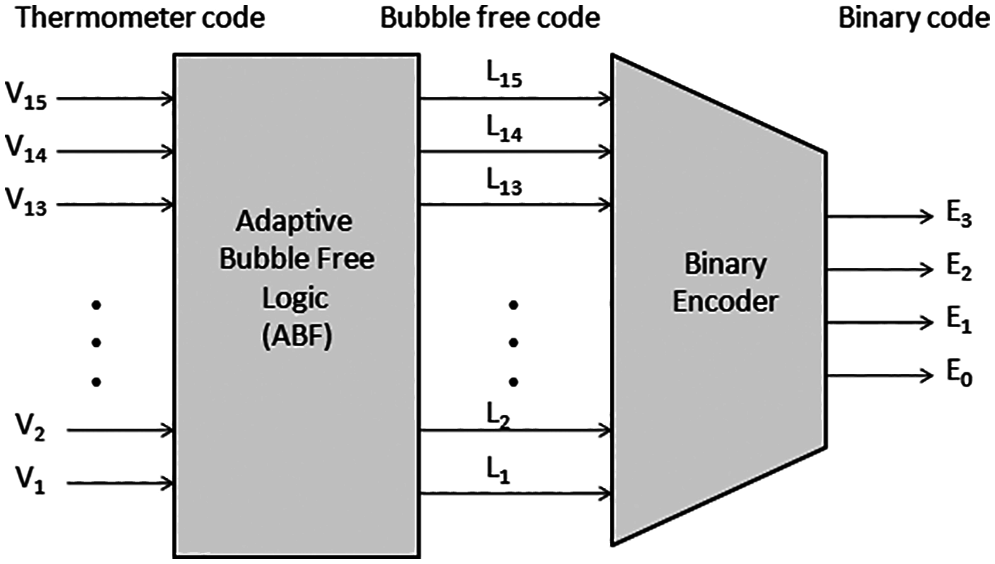Figure 5: Functional blocks of an adaptive bubble free logic with binary encoder

The bubble fault examples in the thermometer code are shown in Tab. 3. There are four types of bubble faults mentioned in that table are 1st order, 2nd order, 3rd order and 4th order. If the 1st order fault identified, in a regular situation, the correct sequence may be 000000000000011 or 000000000001111. Getting 1st order fault in the table, in normal condition, the correct sequence is 000000000000011 or 000000000001111. Nonetheless, the bubble fault happens in the bit V3 for this situation, so it makes the succession frail as 000000000001011. A comparative circumstance occurs with 2nd order, 3rd order and 4th order bubble faults. These bubble faults are corrected by an adaptive bubble fault free circuit.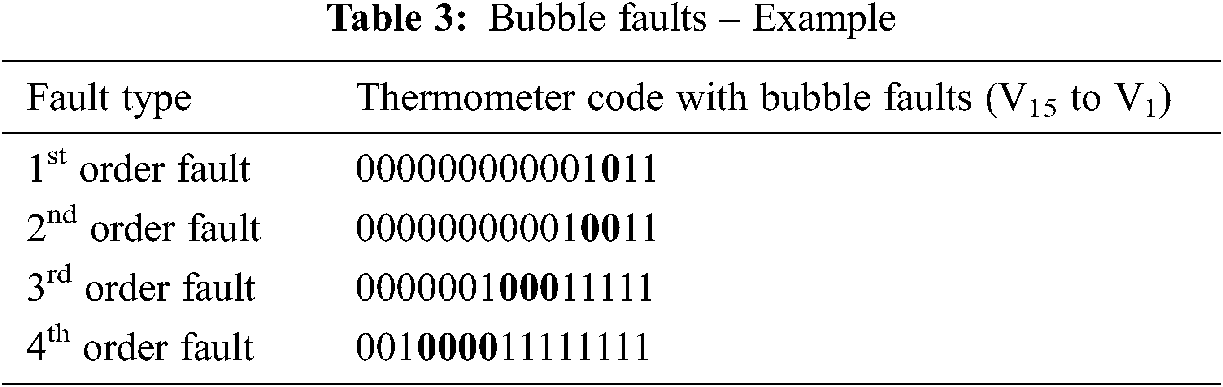Tab. 4 shows the truth table of the Adaptive Bubble Free logic and binary encoder. From this table it is observed that the subsequent code of the CITIQ comparator arrangement named thermometer code with bubble faults is noted by V15 to V1, the outputs of the adaptive bubble free logic circuit is represented by L15 to L1 and the encoded binary output indicated by E3 - E0. The combination of adaptive bubble free logic circuit and binary encoder is linked to accomplish fast over the current encoders, for example, Fat tree structured encoder, ROM encoder, and Multiplexer based encoder and Priority encoder.4  Results and Discussion

S-Edit (Tanner EDA Tool) is used to implement the 4-bit C-FADC schematic in 0.25 µm CMOS Technology, and T-Spice is used to simulate it. The W-Edit displays the output waveforms.

4.1 Simulation Result of CITIQ Comparator

Fig. 6, shows the simulation outcome of a single stage CITIQ Comparator. In Tab. 5, the power utilization and propagation delay for different comparators are evaluated. As compared to Open Loop Comparator, Quantum Voltage Comparator, Threshold Inverter Quantization Comparator, and Improved Threshold Inverter Quantization Comparator, the recommended CITIQ comparator plan reduces the utilization of power by 81.54% 76.52%, 72.72%, and 58.0% respectively. The power utilization and propagation delay evaluation for various comparators is depicted in Fig. 7. From this chart, it is seen that the proposed Clocked ITIQ comparator design decreases the propagation delay by 40.45%, 29.09%, 21.21%, and 17.74% when compared to Open Loop Comparator, Quantum Voltage Comparator, Threshold Inverter Quantization Comparator, and Improved Threshold Inverter Quantization Comparator respectively. Eq. (9) is used to measure the propagation delay, which is given as,

tp=(tplh+tphl)2 (9)where,

tplh - Rising propagation period.

tphl - Falling propagation periodFigure 6: Simulation result of CITIQ comparator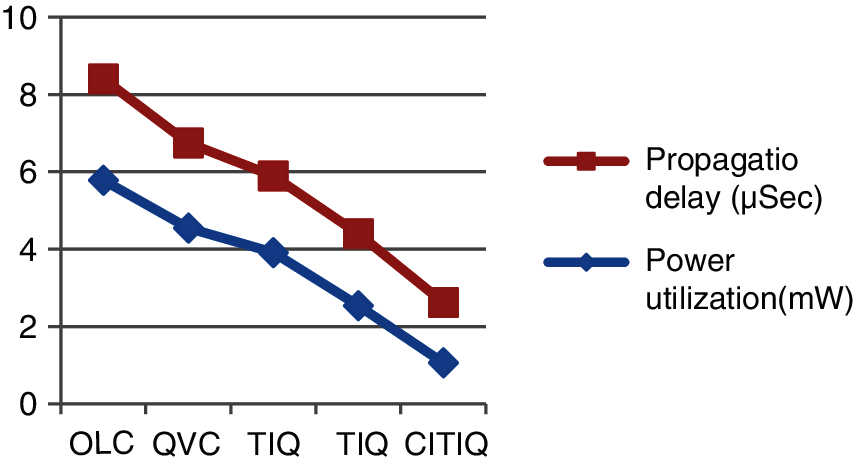Figure 7: Power utilization and propagation retard evaluation of various comparators

4.2 Simulation Result of Binary Encoder and Adaptive Bubble Free Logic Circuit

The power utilization and transistor count comparison of different encoding plans is presented in the Tab. 6. As compared to FTE (Fat Tree Encoder) with Bubble Free circuit, ROME (ROM Encoder) with Bubble Free circuit, MUX-E (Multiplexer based Encoder) with Bubble Free circuit and PE (Priority Encoder) with Bubble Free circuit, the suggested Binary encoder reduces the utilization of power by 76.49%, 74.59%, 65.81%, and 62.8%, and also minimizes area by 44.91%, 44.62%, 42.13% and 36.02%.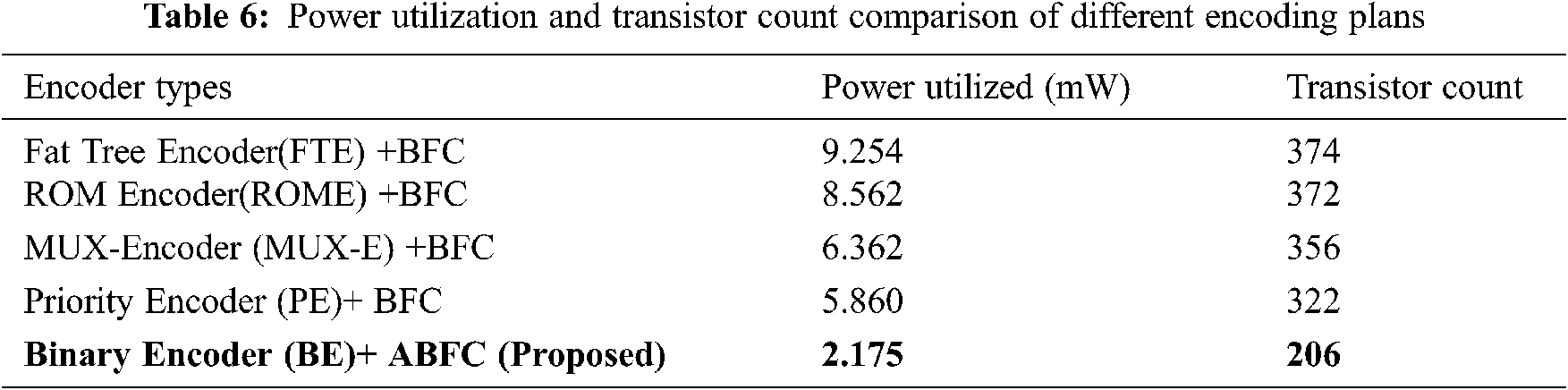4.3 Graphical Response and Outcomes of C-FADC

Fig. 8, shows the graphical response of the Clocked FADC. The 4 Bit Clocked FADC is designed and implemented using Tanner EDA Tool and 250 nm CMOS technology. In terms of area, power utilization, speed, and resolution quality, the Flash ADC performance is examined. The comparison of Power utilization, Reliability and Throughput for different FADCs is listed in Tab. 7. As compared to OLC –FTE ADC, QVC –ROME ADC, TIQ –MUXE ADC, and ITIQ – PE ADC, the desired Clocked FADC brings down the power utilization by 78.45%, 75.28%, 68.45%, and 61.42% less.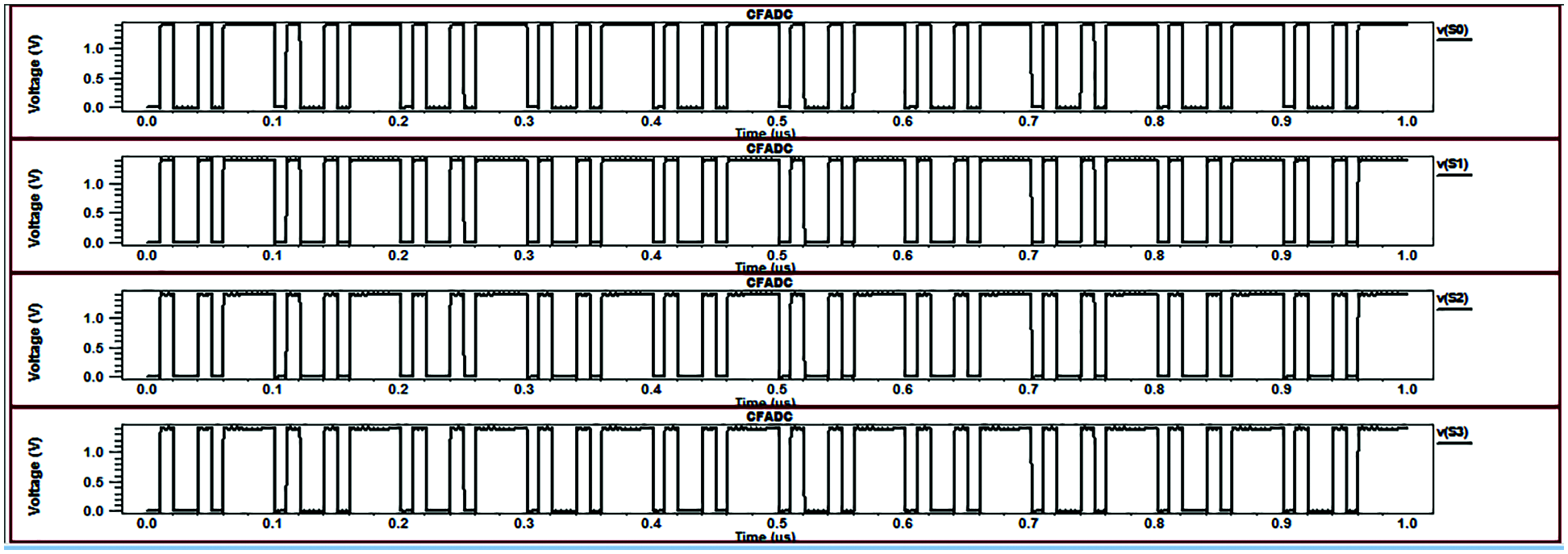Figure 8: Graphical response of clocked FADC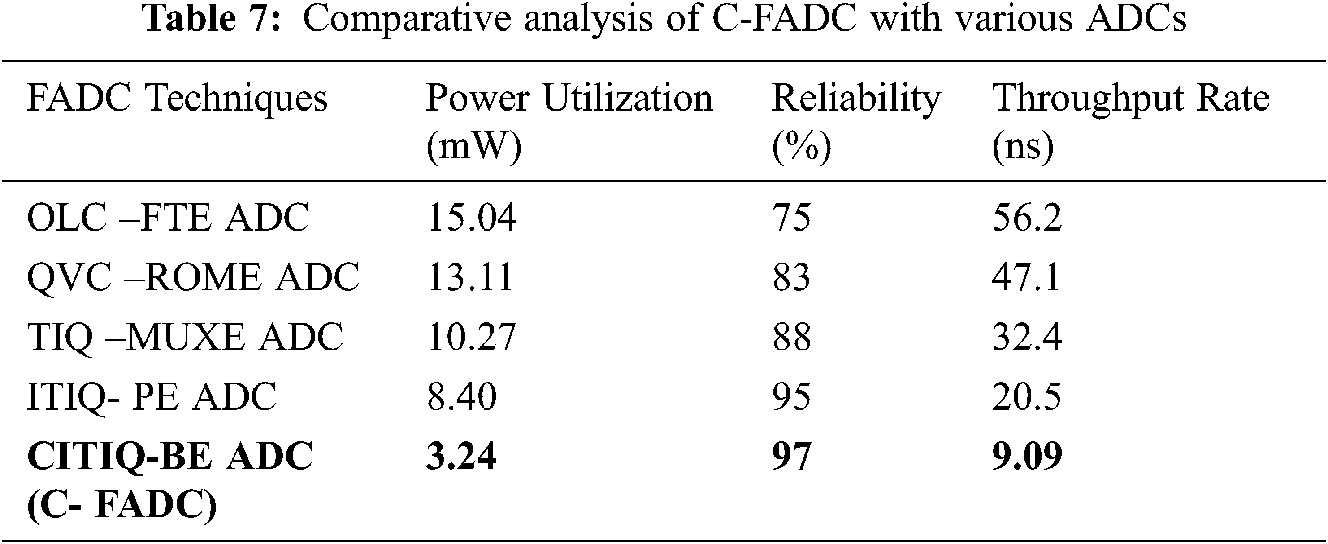When compared to the ITIQ-PE ADC, the proposed CITIQ-BE ADC (C-FADC) achieves a throughput rate of 55.65% and a reliability of 97%. In comparison to the evaluation results, the proposed Clocked FADC outperforms the multiple organized FADCs. Fig. 9, shows the throughput comparisons for various FADC techniques. The DNL and INL results are obtained at 500 MHz Virtual Resolution Bandwidth (VRB), and the deliberate SFDR (Spurious Free Dynamic Range) is 29.3 dB for the input frequency 85 MHz, as noted by the 10th harmonic. The FFT spectrum performance of the C-Flash ADC is shown in Fig. 10 and the SNDR (Signal to Noise Defined Ratio) obtained from that spectrum is 23.2 dB.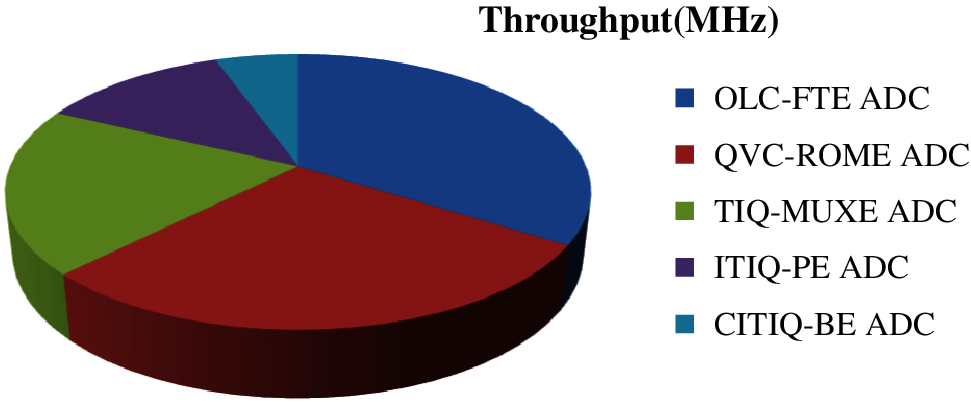Figure 9: Comparison of throughput for different FADC techniquesFigure 10: FFT spectrum output of C-Flash ADC for VRB 500 MHz

The peak differential nonlinearity is +0.65/−0.65 LSB, and the integral nonlinearity is +0.45/−0.5 LSB, as shown in Fig. 11. (i to iv). The proposed FADC utilizes the power is 3.24 mW for a supply potential of 1.4 V and achieves 3.56 effective numbers of bits at 1.2 GS/s. The figure of merit (FOM) is defined as FOM = Power/ (2.VRB.2ENOB). As such, the calculated figure of merit is 0.274 pJ/conv.-step and the summary of static and dynamic parameters of the C-FADC observed from the FFT spectrum is noted down in Tab. 8. The balance between power, speed of execution, and circuit efficiency is clearly surveyed using the Force Delay Product (FDP) thing. When conversion latency is taken into account, the C-FADC arrives at 0.14 pJ - ns/conv. - step, according to the deliberate FDP item.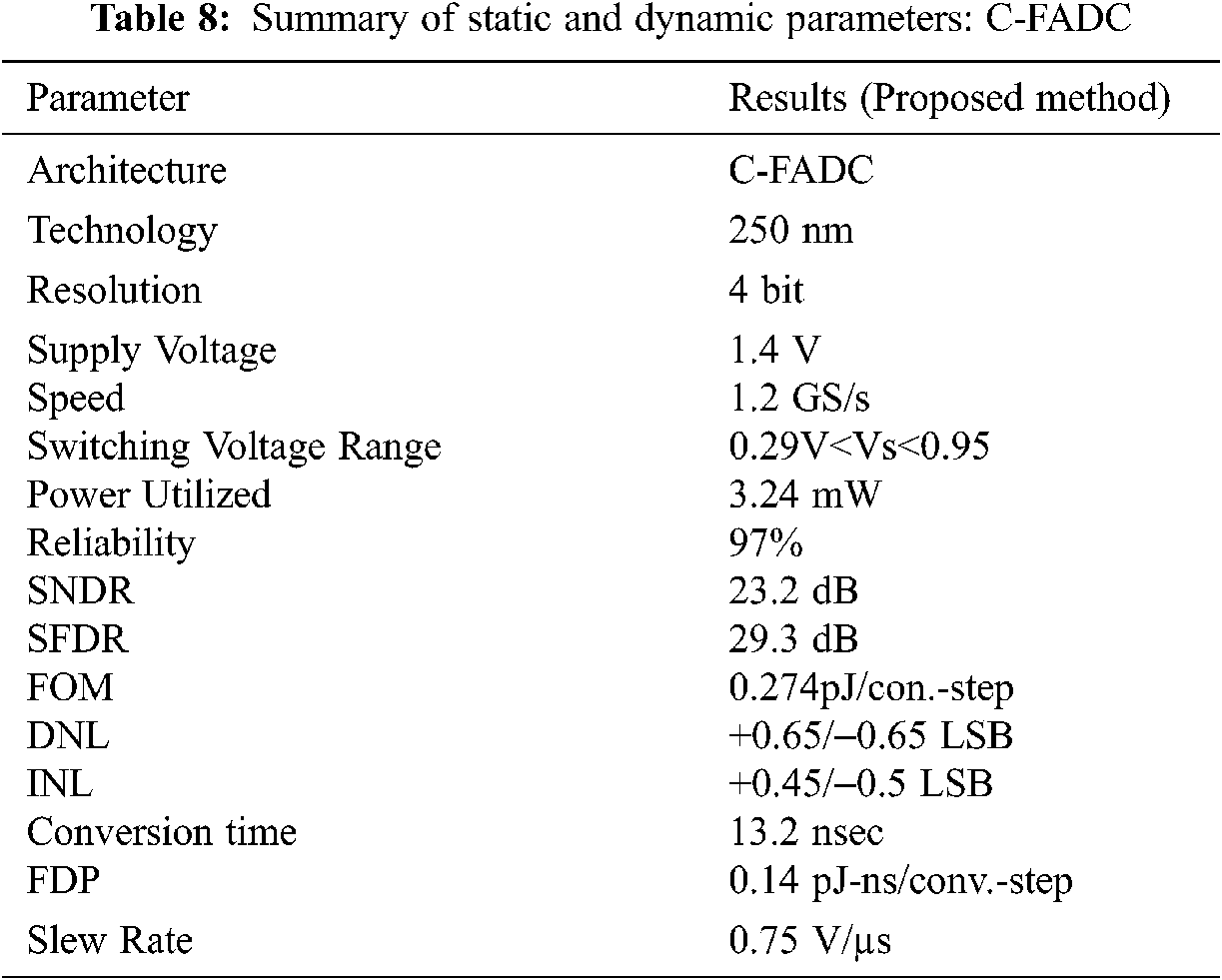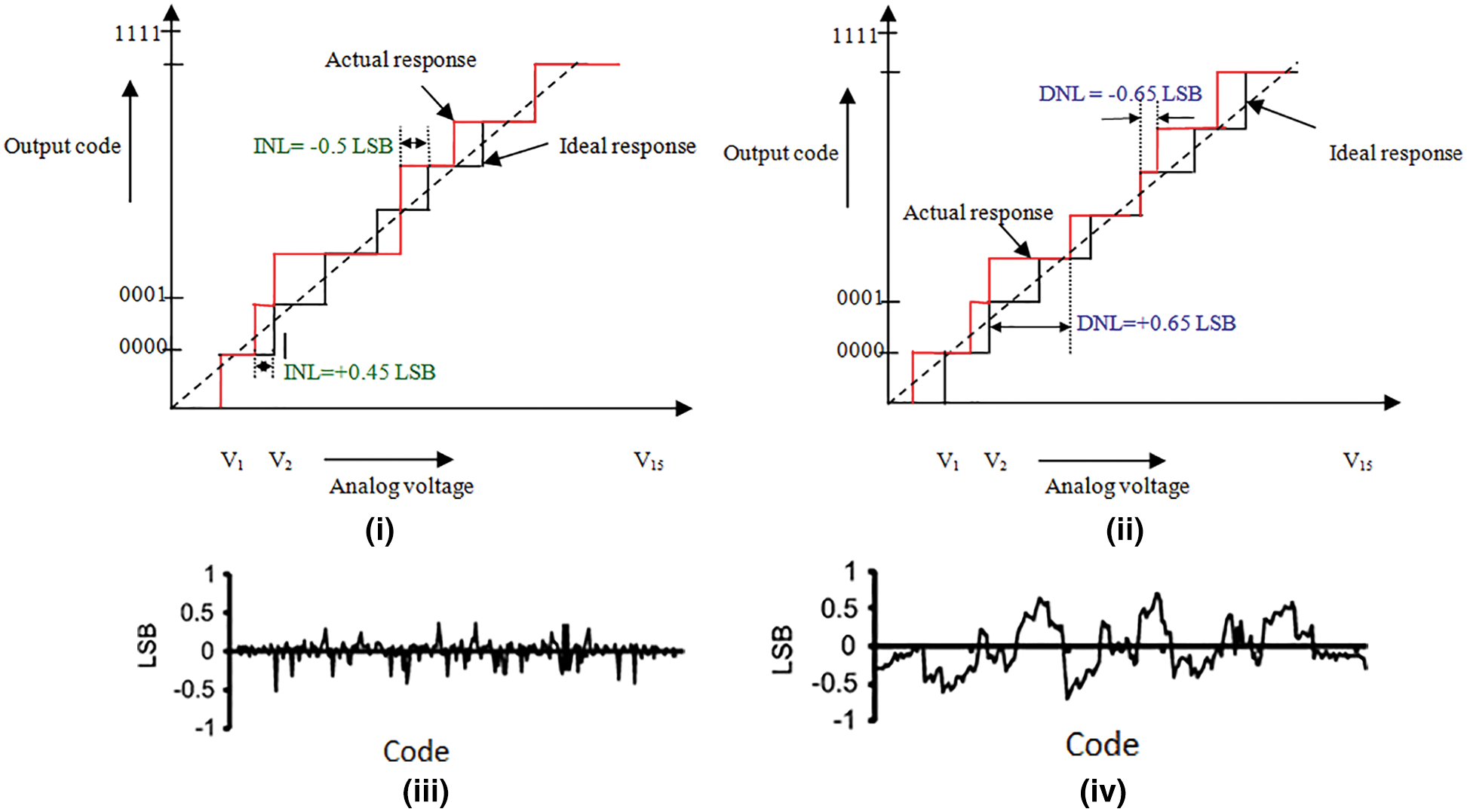Figure 11: (i) and (iii) Integral Non-linearity, (ii) and (iv) Differential Non-Linearity at 500 MHz Virtual resolution bandwidth

The C-FADC results and reference to the state-of-the-art are shown in Tab. 9. It is demonstrated that in FADC architectures, the arranged Clocked Flash ADC can achieve incredible one-of-a-kind execution and a superior balance between Speed, Power, FOM, and VRB. In comparison to the references [1,2,4,7,8,10,11,14,15,19,20,22], the exhibited work uses low power 3.24 mW, and the Figure of Merit (FOM) is lower than the authors [4,8,10,14].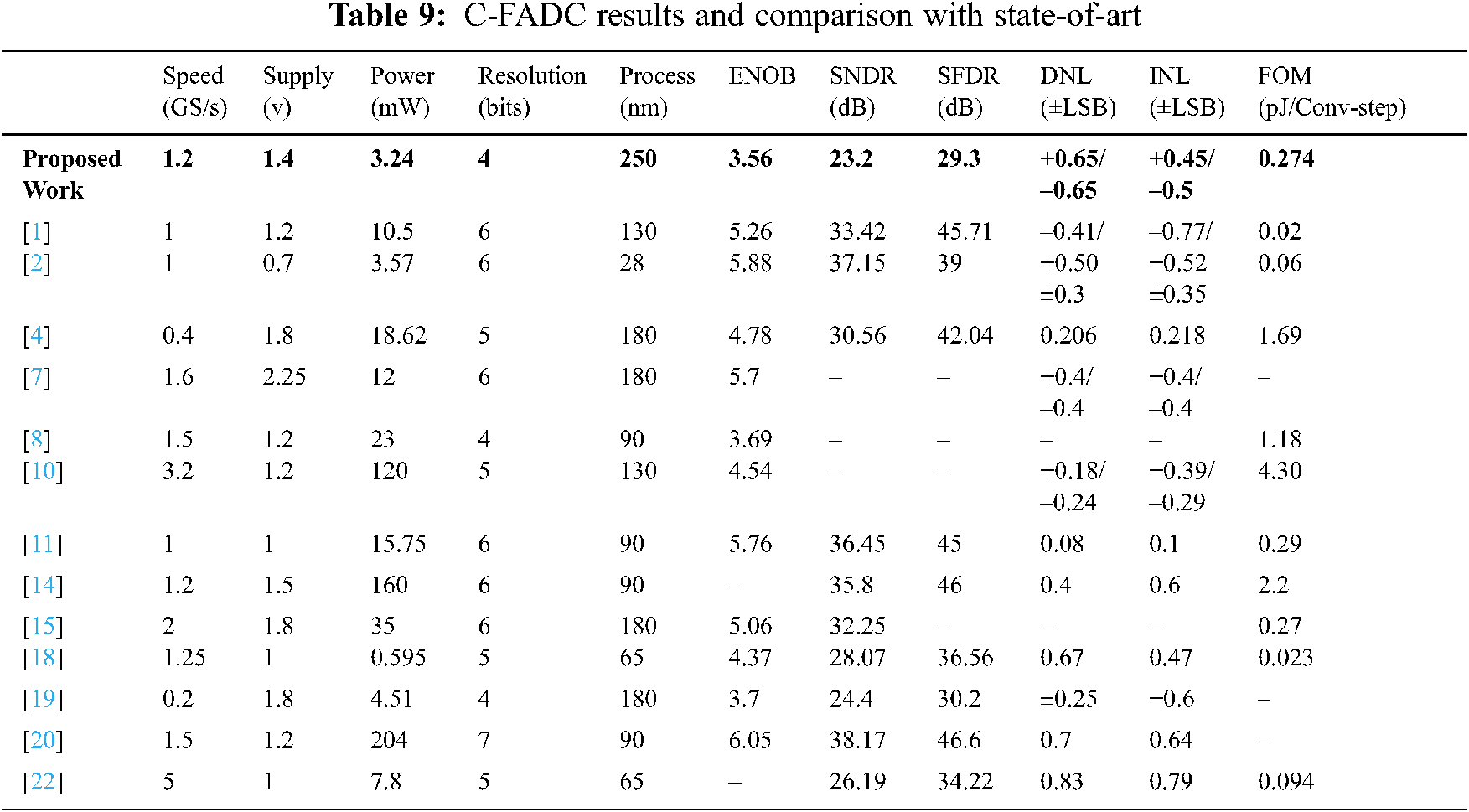5  Conclusion

Tanner EDA is used to build and simulate a 4-bit low-power and high-speed Clocked Flash ADC (C-FADC) that works with 1.4 V input source voltage. From the clocked ITIQ comparator, the clock network diminishes the jitter by minimizing the propagation delay with the switching voltage range is 0.29 V<Vs<0.95 V and achieves the high slew rate is 0.75 V/µs to reduce skew. The adaptive bubble-free logic circuit corrects bubble faults up to fourth order, while the binary encoder transforms the bubble-free code into binary code. With a Virtual Resolution Bandwidth (VRB) of 500 MHz and a speed of 1.2 GS/s, this newly built architecture achieves a FOM of 0.274 pJ/conv.step. The signal to noise distortion ratio is 23.2 dB and spurious free dynamic range is 29.3 dB observed from the FFT-spectrum at 85 MHz input frequency. By using this proposed structure, it is possible to achieve the effective number of bits is 3.56 and the force delay product is 0.14 pJ-ns/conv.-steps. The designed C-FADC structure achieves 97% reliability and has the differential and integral non linearity is ±0.65 LSB and +0.45/−0.5 LSB respectively.

Funding Statement: The authors received no specific funding for this study.

Conflicts of Interest: The authors declare that they have no conflicts of interest to report regarding the present study.

References

1.  S. A. Zahrai and M. Onabajo, “Review of analog-to-digital conversion characteristics and design considerations for the creation of power-efficient hybrid data converters,” Journal of Low Power Electronics and Applications, vol. 8, no. 2, pp. 12–22, 2018.

2.  K. K. Movva and S. Azeemuddin, “A novel low power 6-bit FLASH ADC using charge steering amplifier for RF applications,” in Proc. VDAT, canada, pp. 1–2, 2016.

3.  J. Yoo, K. Choi and J. Ghaznavi, “CMOS flash analog-to-digital converter for high speed and low voltage applications,” in Proc. ACMVLSI, Washington, pp. 56–59, 2003.

4.  S. Khalapure, R. K. Siddharth, Y. K. Nithin Kumar and M. H. Vasantha, “Design of 5-bit flash ADC using multiple input standard cell gates for large input swing,” in Proc. ISVLSI, Bochum, Germany, pp. 585–588, 2017.

5.  R. R. Jogdand, P. K. Dakhole and P. Palsodkar, “Low power flash ADC using multiplexer based encoder,” in Proc. ICIIECS, Coimbatore, India, pp. 1–5, 2017.

6.  A. A. Talukder and M. S. Sarker, “A three-bit threshold inverter quantization based CMOS flash ADC,” in Proc. ICAEE, Dhaka, Bangladesh, pp. 352–356, 2017.

7.  P. C. Scholtens and M. Vertregt, “A 6-b 1.6-Gsample/s flash ADC in 0.18-μm CMOS using averaging termination,” IEEE Journal of Solid-State Circuits, vol. 37, no. 12, pp. 1599–1609, 2002.

8.  T. Sundstrom and A. Alvandpour, “Utilizing process variations for reference generation in a flash ADC,” IEEE Transactions on Circuits and Systems, vol. 56, no. 5, pp. 364–368, 2009.

9.  K. Lokesh Krishna and T. Ramashri, “VLSI Design of 12-bit ADC with 1GSPS in 180 nm CMOS integrating with SAR and two-step flash ADC,” Journal of Theoretical & Applied Information Technology, vol. 68, no. 1, pp. 27–35, 2014.

10. Y. Z. Lin, C. W. Lin and S. J. Chang, “A 5-bit 3.2-GS/s flash ADC with a digital offset calibration scheme,” IEEE Transactions on Very Large Scale Integration (VLSI) Systems, vol. 18, no. 3, pp. 509–513, 2010.

11. K. Lad and M. S. Bhat, “A 1-V 1-GS/s 6-bit low-power flash ADC in 90-nm CMOS with 15.75 mW power consumption,” in proc, ICCCI, Coimbatore, India, pp. 1–4, 2013.

12. B. Van Hieu, S. Choi, J. Seon, Y. Oh, C. Park et al., “A new approach to thermometer-to-binary encoder of flash adcs-bubble error detection circuit,” in Proc. MWSCAS, Seoul, Korea, pp. 1–4, 2011.

13. A. Ozdemir, M. Alrizah and K. Choi, “Optimization of comparator selection algorithm for TIQ flash ADC using dynamic programming approach,” in Proc. ISVLSI, Miami, USA, pp. 495–500, 2019.

14. C. Sandner, M. Clara, A. Santner, T. Hartig and F. Kuttner, “A 6-bit 1.2-GS/s low-power flash-ADC in 0.13-/spl mu/m digital CMOS,” IEEE Journal of Solid-State Circuits, vol. 40, no. 7, pp. 1499–1505, 2005.

15. A. Amini, A. Baradaranrezaeii and M. Hassanzadazar, “A novel online offset-cancellation mechanism in a low-power 6-bit 2GS/s flash-ADC,” Analog Integrated Circuits and Signal Processing, vol. 99, no. 2, pp. 219–229, 2019.

16. Z. Huang and H. Zhang, “Low-voltage bulk-driven high-speed comparator for ADCs,” Electronics Letters, vol. 55, no. 13, pp. 735–737, 2019.

17. L. Chacko and G. Tom Varghese, “Comparator design for low power high-speed flash ADC-a review,” in Proc. IEEE-ICCMC, Erode, India, vol.27, pp. 869–872, 2019.

18. C. H. Chan, Y. Zhu, S. Sin, U. Seng-Pan, R. P. Martins et al., “A 5-bit 1.25-GS/s 4x-capacitive-folding flash ADC in 65-nm CMOS,” IEEE Journal of Solid-State Circuits, vol. 48, no. 9, pp. 2154–2169, 2013.

19. A. Gupta, A. Singh and A. Agarwal, “Highly-digital voltage scalable 4-bit flash ADC,” IET Circuits, Devices & Systems, vol. 13, no. 1, pp. 91–97, 2019.

20. J. Pernillo and M. P. Flynn, “A 1.5-GS/s flash ADC with 57.7-dB SFDR and 6.4-bit ENOB in 90 nm digital CMOS,” IEEE Transactions on Circuits and Systems, vol. 58, no. 12, pp. 837–841, 2011.

21. G. Prathiba, M. Santhi and A. Ahilan, “Design and implementation of reliable flash ADC for microwave applications,” Microelectronics Reliability, vol. 88–90, pp. 91–97, 2018.

22. C. H. Chan, Y. Zhu, S. W. Sin, U. Seng-Pan, R. P. Martins et al., “A 7.8-mW 5-b 5-GS/s dual-edges-triggered time-based flash ADC,” IEEE Transactions on Circuits and Systems, vol. 64, no. 8, pp. 1966–1976, 2017.This work is licensed under a Creative Commons Attribution 4.0 International License, which permits unrestricted use, distribution, and reproduction in any medium, provided the original work is properly cited.